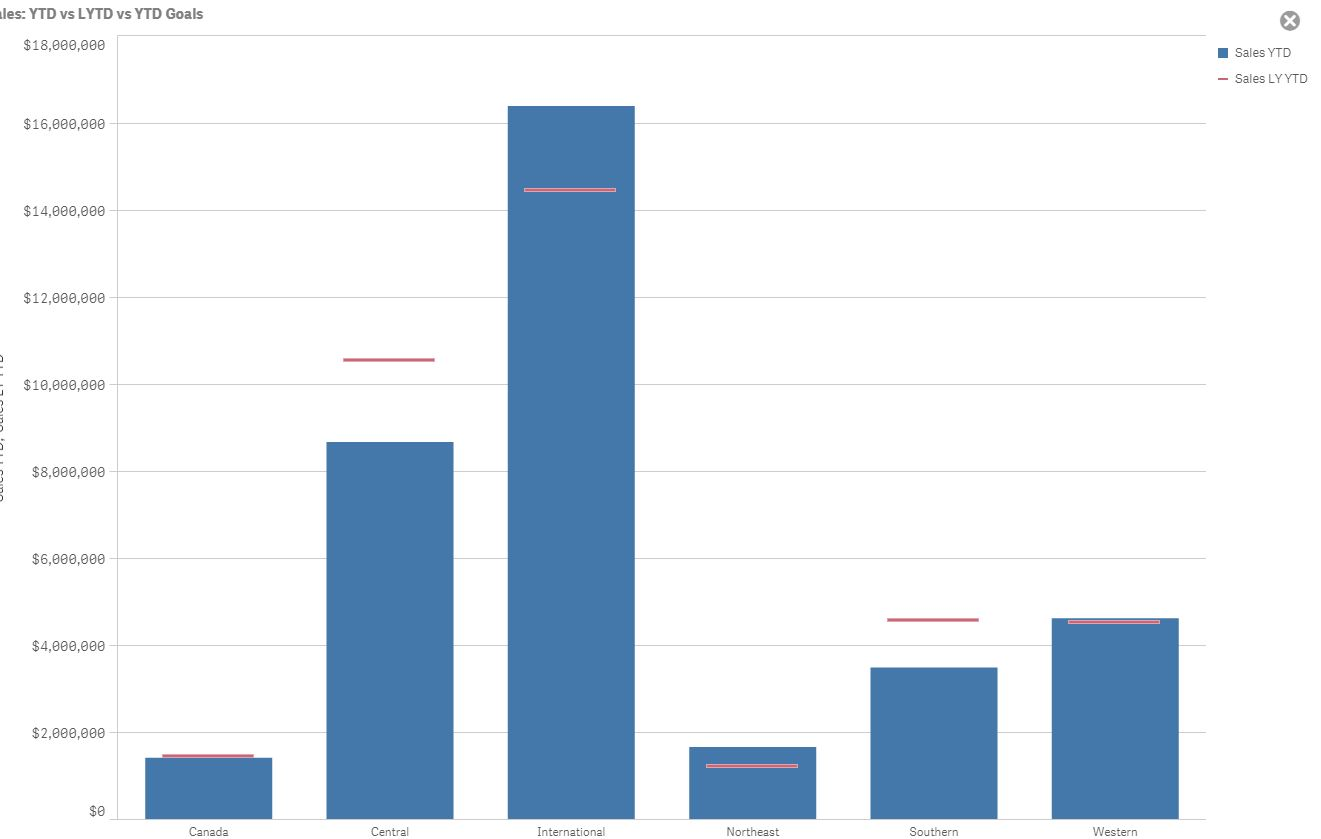# New to Qlik Sense

Discussion board where members can get started with Qlik Sense.

Announcements
BI & Data Trends 2021. Discover the top 10 trends emerging in today. Join us on Dec. 8th REGISTER
cancel
Showing results for
Did you mean:
HighlightedContributor III

## Show sales between 2 dates

I'm a newbie, very newbie so pls be delicate only 2 weeks now

I have sales data for various host channels and need to show sales for This year and the equivalent time period last year.

With some community searching I ended up setting up some variables

vTYear     =Max([Acc Year])

vLYear   =Max([Acc Year])-1  e.g. 2017

vCurrentWk   =FirstSortedValue(distinct [Acc Week],-[Start Wk]) e.g. Wk_26

vCurrentStWk   =Max([Start Wk])  e.g. 24/06/2018

Wanted to set up a variable to show the [Start Wk] date for last year by looking up the equivalent Wk_ number but couldn't figure out the expression

vLYCurrentStWk   e.g. 25/06/2017    I tried this

=date(FirstSortedValue(distinct [Start Wk], aggr({<[Acc Year] = {\$(vLYear)}>}, {<[Acc Week] = {\$(vCurrentWk)}>})))

but it didn't work

Then intended to put a date range into a Set Analysis but again could not figure out the expression

I gave up on Variables and tried this but again no joy

sum({\$<[Start Wk]={">=\$(=date(FirstSortedValue( distinct [Start Wk], [Acc Year]= 2017)))<=\$(=max([Start wk])-365)"}>} [Net GBP incVat])

The table would be similar to this

Start WkAcc YearAcc WeekHostNet GBP incVat
05/02/20172017Wk_06Channel A100
25/06/20172017Wk_26Channel B80
28/04/20182018Wk_05Channel A150
06/05/20182018Wk_19Channel B70
24/06/20182018Wk_26Channel B100

And the results would look similar to this with a line showing the LY YTD salesCan anyone shed some light please

1 Solution

Accepted Solutions
HighlightedMaster II

For YTD maybe you should try

sum({\$< Month = {"<=\$(=num(Month))"} >} [Net GBP incVat])

Here Month field would have values such as Jan,Feb,Mar... etc.

LastYearFlag will always give you previous year which is static and will show 0 for 2016 or 2015...

to make it dynamic (select any year) you can use Year = { \$(=Max(Year) - 1)}

sum({\$< Month = {"<=\$(=num(Month))"},Year = { \$(=Max(Year) - 1)} >} [Net GBP incVat])

Now this will only get you previous years YTD not the current YTD

So use the 2nd expression but by default the value you see without year selection will be last years data and check if you need to add Month or Quarter as extra field for filter selections

Ex: sum({\$< Month = {"<=\$(=num(Month))"},Year = { \$(=Max(Year) - 1)}, Month >} [Net GBP incVat])

If your questions has been resolved, please mark it correct and close it?

Thanks.

3 Replies
HighlightedMaster II

Not sure but...

Use below in your Calendar script...

if(InYear(TempDate, today(),-1), 1, 0) as LastYearFlag,

num(Week(TempDate)) as WeekNum,

WeekStart(TempDate) as WeekStart,

In front end add a filter to setanalysis

sum({\$<LastYearFlag= {1},WeekStart>} [Net GBP incVat])

Maybe this should help?

Lastyear Flag = 1 should always give you previous year, in this case it will be 2017 and if there is data for the week then it would automatically show the data for start week

If you still have trouble try to upload a sample data?

Thanks.

HighlightedContributor III

Thanks Shahbaz

The InYear(TempDate, today(),-1), 1, 0) as LastYearFlag flagged the entire year for some reason not the Year to Date

But I did manage to add the flag to the data source so sum({\$<LastYearFlag= {1}>} [Net GBP incVat]) is now doing the job

thanks for the help and steering me in the right direction

HighlightedMaster II

For YTD maybe you should try

sum({\$< Month = {"<=\$(=num(Month))"} >} [Net GBP incVat])

Here Month field would have values such as Jan,Feb,Mar... etc.

LastYearFlag will always give you previous year which is static and will show 0 for 2016 or 2015...

to make it dynamic (select any year) you can use Year = { \$(=Max(Year) - 1)}

sum({\$< Month = {"<=\$(=num(Month))"},Year = { \$(=Max(Year) - 1)} >} [Net GBP incVat])

Now this will only get you previous years YTD not the current YTD

So use the 2nd expression but by default the value you see without year selection will be last years data and check if you need to add Month or Quarter as extra field for filter selections

Ex: sum({\$< Month = {"<=\$(=num(Month))"},Year = { \$(=Max(Year) - 1)}, Month >} [Net GBP incVat])

If your questions has been resolved, please mark it correct and close it?

Thanks.